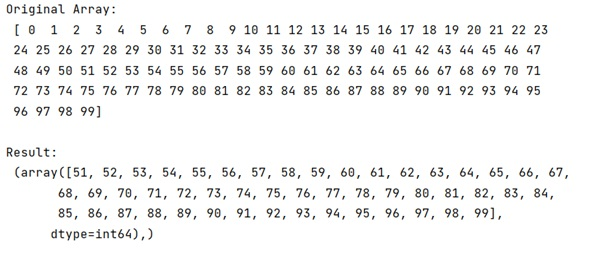# How does python numpy.where() work?

Learn, how does python numpy.where() work in Python?
Submitted by Pranit Sharma, on January 09, 2023

NumPy is an abbreviated form of Numerical Python. It is used for different types of scientific operations in python. Numpy is a vast library in python which is used for almost every kind of scientific or mathematical operation. It is itself an array which is a collection of various methods and functions for processing the arrays.

## Working of numpy.where() method

The numpy.where() method has 2 'operational modes', first one returns the indices, where the condition is True and if optional parameters x and y are present (same shape as condition, or broadcastable to such shape!), it will return values from x when the condition is True otherwise from y. So, this makes where more versatile and enables it to be used more often.

In other words, it returns a tuple of length equal to the dimension of the numpy ndarray on which it is called (in other words ndim) and each item of the tuple is a numpy ndarray of indices of all those values in the initial ndarray for which the condition is True.

Let us understand with the help of an example,

## Python code to demonstrate the example how does numpy.where() work

```# Import numpy
import numpy as np

# Creating an array
arr = np.arange(100)

# Display original array
print("Original Array:\n",arr,"\n")

# Using numpy where
res = np.where(arr > 50)

# Display result
print("Result:\n",res,"\n")
```

Output: